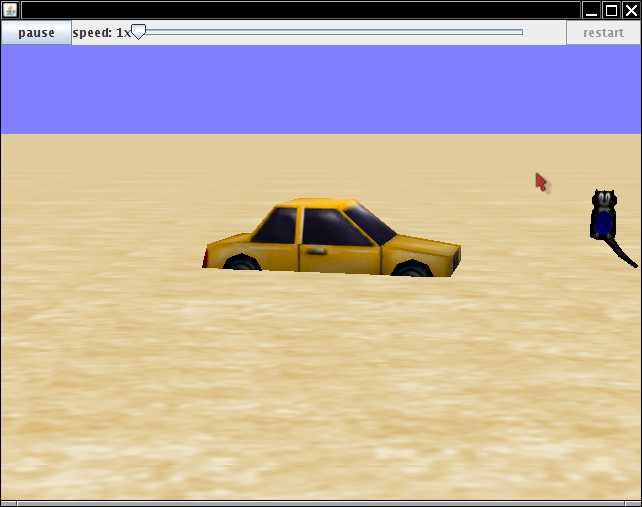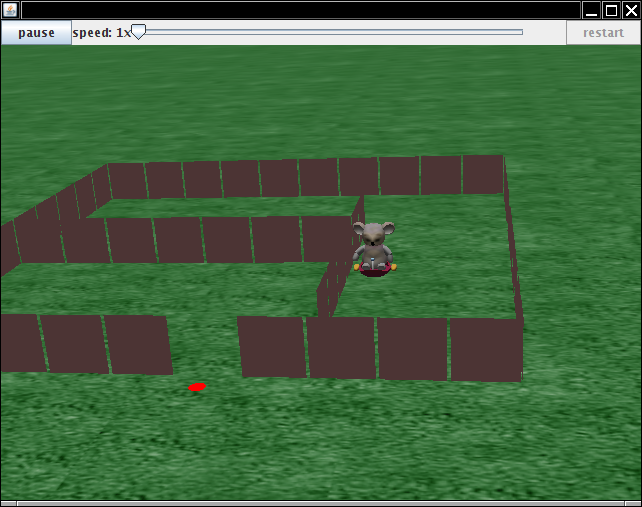# Alice 3, CS1, and Quaternions

This semester, I am teaching the CS1 course again. If you just teach plain Java, it isn't easy to come up with interesting lab assignments. Some of the students have built exciting animations with Alice in the CS0 course. Somehow, they aren't as excited about printing prime numbers or digits of π in CS1. But the latest version of Alice, now in beta, can be programmed in Java. This is very cool. Students can write Java code that directs the Alice models. For example, one assignment asks students to make a `Car` class whose `drive` method moves the car and reduces the gas in the tank. (I couldn't find a gas gauge, so I used the cat clock. The tail moves to the left as the tank gets emptier.) I provide the code for moving the car and rotating the tail, and the students compute the gas consumption.Here is my procedure for making a lab assignment.

• Arrange the objects that students should manipulate in the Alice environment and save the world.
• Open the world in Netbeans (with the Alice 3 plugin)
• Clean up the generated code and make subclasses of the Alice classes, adding fields and methods used for the assignment
• Replace `nbproject/private/private.properties` with an empty file
• Zip up the Netbeans project directory and post for students

The CS1 students use NetBeans, not the Alice IDE. (The Alice IDE is wonderful for pre-CS1 because students drag and drop statements instead of typing them in. They can never make a syntax error! But in CS1, we want to use actual Java, and students need to learn how to deal with syntax errors.) Autocompletion works nicely (and is pretty much a requirement since there is no API documentation available yet). Students learn how to implement methods, branches, and loops in Java. The visual feedback with the Alice models really helps with learning the material.

There are some things that are easier to do with Alice-in-Netbeans than with the Alice IDE, in particular constructing lots of objects. It is a simple matter for the car to drop toasters to mark the path, or to make a whole bunch of wall segments in a maze:There are just a few things you need to know:

1. In the Alice coordinate system, the x-axis runs from left to right, y from bottom to top, and z from front to back. You can hide that from your students, but you need to know yourself when you implement the classes that they extend.
2. To insert another object into the same scene in its default orientation, use
```Transformable obj = new Toaster(); // or some other model
obj.setLocalPointOfView(new PointOfView(
new Quaternion(0, 0, 0, 1),
new Position(x, y, z)));
3. If you want different orientations (such as the walls above), you can call the `turn` method:
`obj.turn(TurnDirection.LEFT, angle, 0);`

Here, 0 means that the turning happens instantaneously, and `angle` is between 0 and 1. (A quarter turn would have an angle of 0.25).

Or, you can use quaternions. In Alice, the quaternion a + b i + c j + d k is a ```new Quaternion(b, c, d, a)```. That is, the real coefficient comes last! In other words, `new Quaternion(0, 0, 0, 1)` is the unit quaternion. Rotation around the vector (x, y, z) with angle α is sin(α/2) (x i + y j + z k) + cos(α/2). For example, to rotate a wall 90 degrees around the y-axis (as with the walls facing east/west in the image above), you use a ```new Quaternion(0, Math.sqrt(0.5), 0, Math.sqrt(0.5))```.

4. If you want to write code for object interactions, use
```for (Composite match : getScene().findAllMatches()) {
if (match instanceof Wall) ...
}```

Of course, you want to hide those quaternions from your students in a suitable superclass, or they will beg you to go back to computing prime numbers or the digits of π.

If you want to give all this a try, download Alice 3 and the Netbeans plugins from the Kenai site and check out the labs that are posted here.